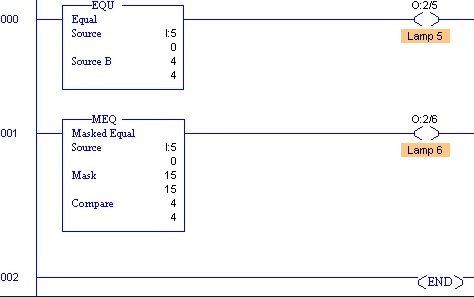# Comparison instructions of PLC### Introduction

Comparison instructions in PLC are used to test pairs of values to condition the logical continuity of a rung. As an example, suppose a LES instruction is presented with two values. If the first value is less than the second, then the comparison instruction is true. To learn more about the compare instructions, we suggest that you read the Compare Instructions Overview that follows.

Equal (EQU)

Use the EQU instruction to test whether two values are equal. If source A and source B are equal, the instruction is logically true. If these values are not equal, the instruction is logically false. Source A must be an address. Source B can either be a program constant or a address. Negative integers are stored in two’s complement form.Not Equal (NEQ)

Use the NEQ instruction to test whether two values are not equal. If source A and source B are not equal, the instruction is logically true. If the two values are equal, the instruction is logically false. Source A must be an address. Source B can be either a program constant or an address. Negative integers are stored in two’s complement form.Less Than (LES)

Use the LES instruction to test whether one value (source A) is less than another (source B). If source A is less than the value at source B, the instruction is logically true. If the value at source A is greater than or equal to the value at source B, the instruction is logically false. Source A must be an address. Source B can either be a program constant or an address. Negative integers are stored in two’s complement form.Less Than or Equal (LEQ)

Use the LEQ instruction to test whether one value (source A) is less than or equal to another (source B). If the value at source A is less than or equal to the value at source B, the instruction is logically true. If the value at source A is greater than the value at source B, the instruction is logically false. Source A must be an address. Source B can either be a program constant or an address. Negative integers are stored in two’s complement form.Greater Than (GRT)

Use the GRT instruction to test whether one value (source A) is greater than another (source B). If the value at source A is greater than the value at source B, the instruction is logically true. If the value at source A is less than or equal to the value at source B, the instruction is logically false. Source A must be an address. Source B can either be a program constant or an address. Negative integers are stored in two’s complement form.Greater Than or Equal (GEQ)

Use the GEQ instruction to test whether one value (source A) is greater than or equal to another (source B). If the value at source A is greater than or equal to the value at source B, the instruction is logically true. If the value at source A is less than the value at source B, the instruction is logically false. Source A must be an address. Source B can either be a program constant or an address. Negative integers are stored in two’s complement form.Use the MEQ instruction to compare data at a source address with data at a compare address. Use of this instruction allows portions of the data to be masked by a separate word.Limit Test (LIM)

Use the LIM instruction to test for values within or outside a specified range, depending on how you set the limits.